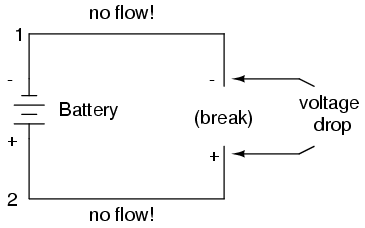# Why does the full PD across a battery manifest itself across a break in a circuit at any point?

Consider the following circuit:I want to know why the full pd of the battery can be measured across the break if a voltmeter was connected. Surely when the whole circuit was closed the charges at the start of the break have less electrical potential than charges right next to the negative end of the battery, and charges at the end of the break have more potential than charges at the positive end of the battery, so they cannot have the same voltage as the battery.

In a static situation there is no electric field inside a conductor, otherwise the charges would redistribute until they stop moving (and thus the electric field is zero). By a similar reasoning, the electric field in the surface of a conductor is normal to the surface.

A conductor is thus an equipotential surface, the potential is the same all along the conductor. If you have two wires connected to the two ends of the battery, then each will be at the same potential as the end of the battery to which it is connected.

You are right that at the very initial moment, where the wire pieces are attached to the battery terminals, they are at a different potential than the terminals themselves.

But that equalizes itself in a blink of an eye.

• The negative battery terminal is stuffed with negative charges. They all repel each other. We call this high potential.

• When you attach a wire piece to this terminal then suddenly these charges have another place to go where the repulsion is smaller (where the potential is lower). So they move there. The potential falls on the wire.

• As they leave the terminal, the battery carries new negative charge to the terminal continously so it always is at the same potential. This new negative charge will then also move away.

• This continues until just as much charge is located at the wire piece as is at the terminal. Only then will the charges stop moving. If there was a difference, then the repulsions would be different (the potentials would be difference), and the charge wound still be moving. Only when the charge accumulations are the same (when the potentials are equal) will the chare stop flowing.

The same goes for the positive terminal, just with a lack of charge and thus attraction rather than repulsion. Since the battery always keeps its terminals at a specific potential, the above explanation tells why the attached wire pieces will get that same potential.

You two wires with a break across them are equivalent to a capacitor with a very small capacitance $$C$$. The time constant of the circuit is $$CR$$ where $$R$$ is the resistance of the circuit and also probably dominated by the internal resistance of the battery.
It will therefore not take very much time for the circuit to settle down to a steady state configuration (no current flowing in the circuit and hence no drop in potential across the resistance of the wires and the battery) with the capacitor fully charged the potential difference across it will equal the emf of the battery.

If you connect a voltmeter across the braek a current will flow in the circuit and the potential difference measured by the voltmeter will be less than the emf of the battery due to the resistance of the wires and the cell. If the resistance of the voltmeter is very much higher than the resistance of the wires and the battery then the reading will be, to a very good approximation, equal to the emf of the battery.